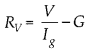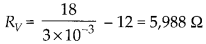Enlightened

# A galvanometer coil has a resistance of 12 Q and the metre shows full scale deflection for a current of 3 mA. How will you convert metre into a voltmeter of range 0 to 18 V?

• 0

A galvanometer coil has a resistance of 12 Q and the metre shows full scale deflection for a current of 3 mA. How will you convert metre into a voltmeter of range 0 to 18 V?

Share

1. Solution:
By using the formulaWe can calculate required resistance to be connected in series.Check the complete chapter with solutions.

NCERT Solutions for 12th Class Physics: Chapter 4-Moving Charges and Magnetism

• 0
2. Solution:
By using the formulaWe can calculate required resistance to be connected in series.Check the complete chapter with solutions.

NCERT Solutions for 12th Class Physics: Chapter 4-Moving Charges and Magnetism

• 0